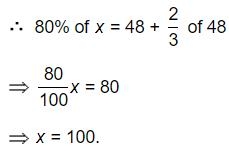Courses

# Test: Percentages- 1

## 10 Questions MCQ Test IBPS PO Mains - Study Material, Online Tests, Previous Year | Test: Percentages- 1

Description
This mock test of Test: Percentages- 1 for Quant helps you for every Quant entrance exam. This contains 10 Multiple Choice Questions for Quant Test: Percentages- 1 (mcq) to study with solutions a complete question bank. The solved questions answers in this Test: Percentages- 1 quiz give you a good mix of easy questions and tough questions. Quant students definitely take this Test: Percentages- 1 exercise for a better result in the exam. You can find other Test: Percentages- 1 extra questions, long questions & short questions for Quant on EduRev as well by searching above.
QUESTION: 1

### Rajeev buys good worth Rs. 6650. He gets a rebate of 6% on it. After getting the rebate, he pays sales tax @ 10%. Find the amount he will have to pay for the goods.

Solution: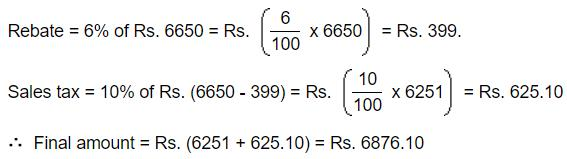QUESTION: 2

### 30% of the men are more than 25 years old and 80% of the men are less than or equal to 50 years old. 20% of all men play football. If 20% of the men above the age of 50 play football, what percentage of the football players are less than or equal to 50 years?

Solution:

Let total number of men = 100
Then
80 men are less than or equal to 50 years old
(Since 80% of the men are less than or equal to 50 years old)
=> 20 men are above 50 years old (Since we assumed total number of men as 100)
20% of the men above the age of 50 play football
⇒ Number of men above the age of 50 who play football =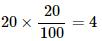Number of men who play football = 20 (Since 20% of all men play football)
Percentage of men who play football above the age of 50 = 4/20 x 100 = 20%
=>Percentage of men who play football less than or equal to the age 50 = 100%−20%=80%

QUESTION: 3

### As a percentage of P is equal to P as a percentage of (P + Q). Find Q as a percentage of P.

Solution:QUESTION: 4

On my sister's 15th birthday, she was 159 cm in height, having grown 6% since the year before. How tall was she the previous year?

Solution:

Given that height on 15th birthday = 159 cm and growth = 6%
Let the previous year height = x
Then height on 15th birthday =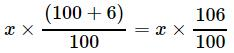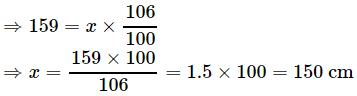QUESTION: 5

If the price of petrol increases by 25% and Benson intends to spend only an additional 15% on petrol, by how much % will he reduce the quantity of petrol purchased?

Solution:

Assume that the initial price of 1 Litre petrol = Rs.100 ,Benson spends Rs.100 for petrol,
such that Benson buys 1 litre of petrol
After the increase by 25%, price of 1 Litre petrol =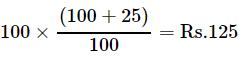Since Benson spends additional 15% on petrol,
amount spent by Benson =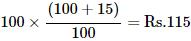Hence Quantity of petrol that he can purchase = 115/125 Litre
Quantity of petrol reduced =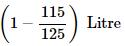Percentage Quantity of reduction =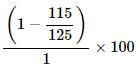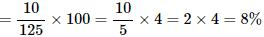QUESTION: 6

A student multiplied a number by 3/5 instead of 5/3. What is the percentage error in the calculation?

Solution:

Let number is 100.
Actual Multiplication = (100 *5)/3 = 500/3.
Student multiplied = (100 *3)/5 = 300/5.
Error,
= (500/3) - (300/5)
= (2500 - 900)/15
= 1600/15
%Error = (1600 *100 *3)/(15*500) = 64%.

QUESTION: 7

The population of a town increased from 1,75,000 to 2,62,500 in a decade. The average percent increase of population per year is:

Solution: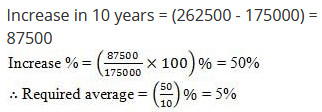QUESTION: 8

Arun got 30% of the maximum marks in an examination and failed by 10 marks. However, Sujith who took the same examination got 40% of the total marks and got 15 marks more than the passing marks. What were the passing marks in the examination?

Solution:

Let x is the maximum marks of the examination
Marks that Arun got = 30 % of x =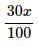Given that Arun failed by 10 marks
⇒ Minimum Pass Mark =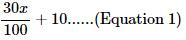Marks that Sujith got = 40 % of x =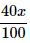Given that Sujith got 15 marks more than the passing marks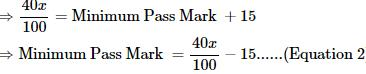From equations 1 and 2, we have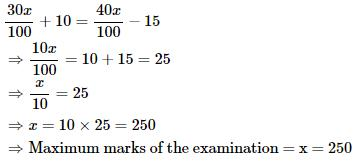Substituting the value of x in Equation 1, we have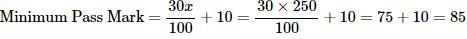QUESTION: 9

P is able to do a piece of work in 15 days and Q can do the same work in 20 days. If they can work together for 4 days, what is the fraction of work left?

Solution:

1day's work of p=1/15

1day's work of q=1/20

1day's work of both=7/60

4days work=7/15

remaining work=1-7/15=8/15

QUESTION: 10

In a certain school, 20% of students are below 8 years of age. The number of students above 8 years of age is 2/3 of the number of students of 8 years of age which is 48. What is the total number of students in the school?

Solution:

Let the number of students be x. Then,
Number of students above 8 years of age = (100 - 20)% of x = 80% of x.